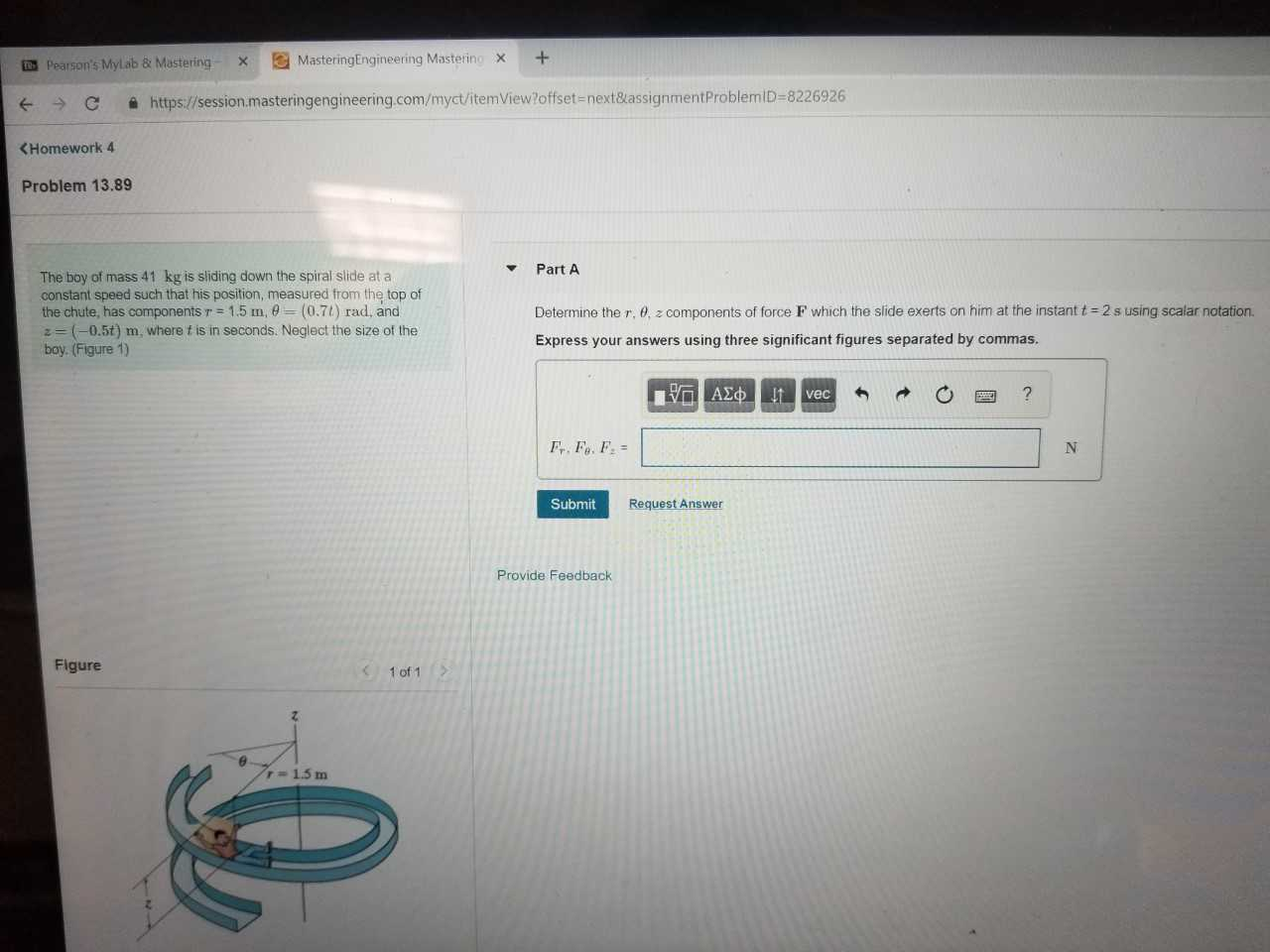# ㅮ Pearson's MyLab & Mastering- × 圏MasteringEngineering Master in、 С https://session.masteringengineering.com/myct/item View?offset next&assignmentProblemlD-8226926 KHomework 4 Problem 13.89 ▼ Part A The boy of mass 41 kg is sliding down the spiral slide at a constant speed such that his position, measured from the top of the chute, has components-: 1.5 m, θ = (0.71 ) rad, and z= (-0.5t) m, where t is in seconds. Neglect the size of the boy. (Figure 1) Determine the r, θ, 2 components of force F which the slide exerts on him at the instant t Express your answers using three significant figures separated by commas 2s using scalar notation. Submit Request Answer Provide Feedback Figure 1 of 12 r=1.5 m

Questionhelp_outlineImage Transcriptioncloseㅮ Pearson's MyLab & Mastering- × 圏MasteringEngineering Master in、 С https://session.masteringengineering.com/myct/item View?offset next&assignmentProblemlD-8226926 KHomework 4 Problem 13.89 ▼ Part A The boy of mass 41 kg is sliding down the spiral slide at a constant speed such that his position, measured from the top of the chute, has components-: 1.5 m, θ = (0.71 ) rad, and z= (-0.5t) m, where t is in seconds. Neglect the size of the boy. (Figure 1) Determine the r, θ, 2 components of force F which the slide exerts on him at the instant t Express your answers using three significant figures separated by commas 2s using scalar notation. Submit Request Answer Provide Feedback Figure 1 of 12 r=1.5 m fullscreen

### Want to see the step-by-step answer?